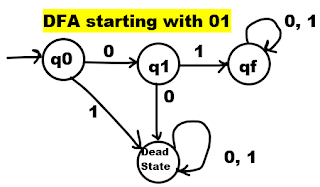# RGPV TOC What do you understand by DFA how to represent it

#### RGPV 2015,14,02,03Q. What do you understand by DFA (Deterministic Finite Automata) and how is it represented ?

Ans. A DFA means Deterministic finite automata or a finite state automata or a deterministic finite state machine (DFSM) or deterministic finite acceptor (DFA).
It is a 5 tuple machine,
M = (Q, Σ, δ, q0, F)
1. Q is a finite non empty set of states.
2. Σ is a finite non empty set of input symbols.
3. δ is a transition function, QXΣ int to Q
4. q0 is an initial state belong to Q.
5. F is the set of final states belong to Q.
Lets take and example to under statnd DFA,
Construct a DFA for the language accepting strings starting with ‘01’ over input alphabets ∑ = {0, 1}.
Sol. Examples of strings accepted,
• 01
• 0101
• 01000000
• 01111111, etc
Here 01 consist 2 characters, so length is 2.
So minimum number of states required = 2+1 = 3.